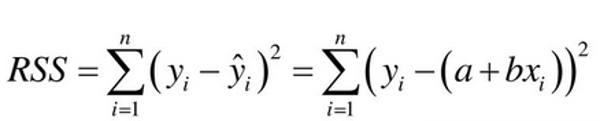Related Tags

statistics
data science
regression

# The residual sum of squaresEducative Answers Team

A residual sum of squares (RSS), also known as the sum of squared residuals (SSR), is a statistical technique used to measure the amount of variance in a data set that is not explained by a regression model. It is the sum of the squared values of the residuals (deviations of predicted from actual empirical values of data).$y_i$ is the $i^{th}$ actual value from data, $\hat{y_i}$ is the $i^{th}$ predicted value using regression, and $a$ and $b$ are constants.

In the graph shown, residualthe difference between the predicted and actual value is represented by the yellow lines.

SSR is the total sum of the squares of these residuals (yellow lines).## Example

Given the set of values:

$X$ $Y$
0 1
1 2
2 6
3 7
4 8

and with $a=1$ and $b=2$, we will get the following result:

$RSS = (1-(1+(2*0)))^2+ (2-(1+(2*1)))^2 + (6-(1+(2*2)))^2 + (7-(1+(2*3)))^2 + (8-(1+(2*4)))^2$

$RSS = 3$

RELATED TAGS

statistics
data science
regression